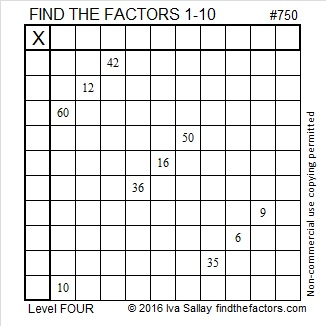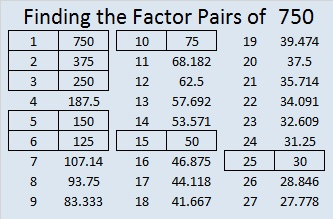# 750 and Level 4

Contents

### Today’s Puzzle:Print the puzzles or type the solution on this excel file: 10 Factors 2016-01-18

Some possible steps to solve the puzzle:### Factors of 750:

• 750 is a composite number.
• Prime factorization: 750 = 2 x 3 x 5 x 5 x 5, which can be written 750 = 2 x 3 x (5^3)
• The exponents in the prime factorization are 1, 1, and 3. Adding one to each and multiplying we get (1 + 1)(1 + 1)(3 + 1) = 2 x 2 x 4 = 16. Therefore 750 has exactly 16 factors.
• Factors of 750: 1, 2, 3, 5, 6, 10, 15, 25, 30, 50, 75, 125, 150, 250, 375, 750
• Factor pairs: 750 = 1 x 750, 2 x 375, 3 x 250, 5 x 150, 6 x 125, 10 x 75, 15 x 50, or 25 x 30
• Taking the factor pair with the largest square number factor, we get √750 = (√25)(√30) = 5√30 ≈ 27.386127875.### Sum-Difference Puzzles:

30 has four factor pairs. One of those pairs adds up to 13, and another one subtracts to 13. Put the factors in the appropriate boxes in the first puzzle.

750 has eight factor pairs. One of the factor pairs adds up to ­65, and a different one subtracts to 65. If you can identify those factor pairs, then you can solve the second puzzle!The second puzzle is really just the first puzzle in disguise. Why would I say that?

### More reasons to be interested in the number 750:

750 can be written as the sum of consecutive numbers seven ways:

• 249 + 250 + 251 = 750; that’s 3 consecutive numbers.
• 186 + 187 + 188 + 189 = 750; that’s 4 consecutive numbers.
• 148 + 149 + 150 + 151 + 152 = 750; that’s 5 consecutive numbers.
• 57 + 58 + 59 + 60 + 61 + 62 + 63 + 64 + 65 + 66 + 67 + 68 = 750; that’s 12 consecutive numbers.
• 43 + 44 + 45 + 46 + 47 + 48 + 49 + 50 + 51 + 52 + 53 + 54 + 55 + 56 + 57 = 750; that’s 15 consecutive numbers.
• 28 + 29 + 30 + 31 + 32 + 33 + 34 + 35 + 36 + 37 + 38 + 39 + 40 + 41 + 42 + 43 + 44 + 45 + 46 + 47 = 750; that’s 20 consecutive numbers.
• 18 + 19 + 20 + 21 + 22 + 23 + 24 + 25 + 26 + 27 + 28 + 29 + 30 + 31 + 32 + 33 + 34 + 35 + 36 + 37 + 38 + 39 + 40 + 41 + 42 = 750; that’s 25 consecutive numbers.

750 is also the sum of all the prime numbers from 17 to 79. Do you remember what those sixteen prime numbers are?

Because 5, 25, and 125 are its factors, 750 is the hypotenuse of three Pythagorean triple triangles:

• 210² + 720² = 750²
• 264² + 702² = 750²
• 450² + 600² = 750²

750² = 562500 which is another cool looking square number whose digits include 5 and the value of 5^4.

750 is also the sum of three squares six different ways:

• 26² + 7² + 5² = 750
• 25² + 11² + 2² = 750
• 25² + 10² + 5² = 750
• 23² + 14² + 5² = 750
• 23² + 11² + 10² = 750
• 19² + 17² + 10² = 750

Wikipedia tells us that 750 is the 15th nonagonal number because 15(7⋅15 – 5)/2 = 750. It is also 10 times the 5th nonogonal number because 10 ⋅ 5(7⋅5 – 5)/2 = 750.

750 is a palindrome in three different bases:

• 23232 BASE 4; note that 2(4^4) + 3(4^3) + 2(4^2) + 3(4^1) + 2(4^0) = 750.
• 2A2 BASE 17 (A= 10 base 10); note that 2(17²) + 10(17) + 2(1) = 750.
• PP BASE 29 (P = 25 base 10); note that 25(29) + 25(1) = 750.

This site uses Akismet to reduce spam. Learn how your comment data is processed.